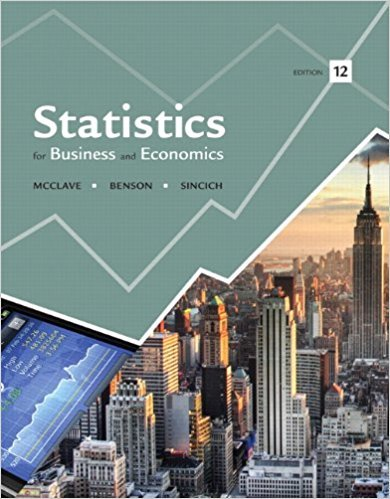×
Get Full Access to Statistics For Business And Economics - 12 Edition - Chapter 5 - Problem 5e
Get Full Access to Statistics For Business And Economics - 12 Edition - Chapter 5 - Problem 5e

×

# Refer to Exercise 5.3. Assume that a random sample of n =ISBN: 9780321826237 51

## Solution for problem 5E Chapter 5

Statistics for Business and Economics | 12th Edition

• Textbook Solutions
• 2901 Step-by-step solutions solved by professors and subject experts
• Get 24/7 help from StudySoup virtual teaching assistantsStatistics for Business and Economics | 12th Edition

4 5 1 365 Reviews
31
0
Problem 5E

Problem 5E

Refer to Exercise 5.3. Assume that a random sample of n = 2 measurements is randomly selected from the population.

a. List the different values that the sample median m may assume and find the probability of each. Then give the sampling distribution of the sample median.

b. Construct a probability histogram for the sampling distribution of the sample median and compare it with the probability histogram for the sample mean (Exercise 5.3, part b).Step-by-Step Solution:

Solution :

Step 1 of 2:

We assume that a random sample of n=2 measurements is randomly selected from the population.

Our goal is :

a). We need to list the different values that the sample median and we have to find the probability

of each.

b). We need to construct histogram for the sampling distribution of the sample median and compare it with the probability histogram for the sample mean.

a). Now we have to list the different values that the sample median

Then we have to find the probability of each. Then give the sampling distribution of the sample median.

We know that sample size n is 2.

Then the sample median table is given below.

 Sample Observation 1 Observation 2 Median (m) Probability 1 1 1 1 0.04 2 1 2 1.5 0.06 3 1 3 2 0.04 4 1 4 2.5 0.04 5 1 5 3 0.02 6 2 1 1.5 0.06 7 2 2 2 0.09 8 2 3 2.5 0.06 9 2 4 3 0.06 10 2 5 3.5 0.03 11 3 1 2 0.04 12 3 2 2.5 0.06 13 3 3 3 0.04 14 3 4 3.5 0.04 15 3 5 4 0.02 16 4 1 2.5 0.04 17
Step 2 of 1

##### ISBN: 9780321826237

The answer to “Refer to Exercise 5.3. Assume that a random sample of n = 2 measurements is randomly selected from the population.a. List the different values that the sample median m may assume and find the probability of each. Then give the sampling distribution of the sample median.b. Construct a probability histogram for the sampling distribution of the sample median and compare it with the probability histogram for the sample mean (Exercise 5.3, part b).” is broken down into a number of easy to follow steps, and 73 words. The full step-by-step solution to problem: 5E from chapter: 5 was answered by , our top Business solution expert on 07/21/17, 05:42AM. Statistics for Business and Economics was written by and is associated to the ISBN: 9780321826237. This full solution covers the following key subjects: sample, median, Probability, exercise, distribution. This expansive textbook survival guide covers 15 chapters, and 1631 solutions. This textbook survival guide was created for the textbook: Statistics for Business and Economics , edition: 12. Since the solution to 5E from 5 chapter was answered, more than 548 students have viewed the full step-by-step answer.

Unlock Textbook Solution